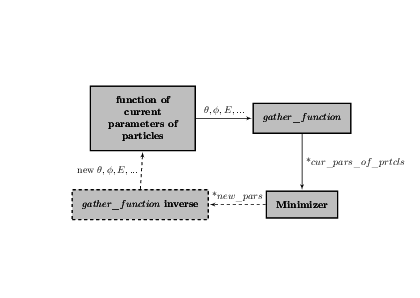# Numerical Minimization with ROOT

I see that ROOT provides many different methods for numerical minimization and it’s awesome.
But unfortunately I can find just examples of using things like `ROOT::Math::GSLMinimizer` and pieces of description. I have two questions. The first is
Where can I find solid information about this method (e.g. I need more details about the output of method `X()`).

And the second.
Suppose I have a function which I want to minimize:

``````double Chi2( const double *current_pars_of_particles )
{
//Here Chi2 will be expressed
//in terms of current_pars_of_particles
return Chi2;
}
``````

And suppose the array `current_pars_of_particles` was obtained from some pre-defined function, which is gathering together parameters of several particles (such that spherical angles, momentums and so on).

``````ROOT::Math::Minimizer* min =
...
min->Minimize();
...
const double *xs = min->X();
``````

am I able to get after that not some new array but just changed `current_pars_of_particles`? Picture below may help.

In other words, I want to just change my initial values. This is desirable because of difficulty which appears when one wants to construct inverse `gather_function` in order to regather (sorry for my English) all parameters back.

I do not how to scale that picture. Sorry.

May be @moneta can tell you more

Hi,

I am not sure I have understood your questions. Your Minimisation algorithm, whatever you use in ROOT, will require a function of a vector of variables x[], the objective function. In your case the function is chi2 and the variables are current_pars_of_particles .

The minimisation algorithm will find the minimum of the function and will return in Minimizer::X() a pointer to the vector containing the variable values at the minimum.

If your variables are actually functions of other variables, and you are interested to these second ones, either you compute the final values yourself or you re-parametrixe your objective function as function of these other variables.

Best Regards

Lorenzo

What I want is something like this:where `*new pars` is pointer to a vector after `minX()` call.
And I do not want to use the gather_function inverse block here or something similar. I.e. I want Minimizer to change my initial variables and not create new. Is this possible?

Hi,

The Minimizer will just call the objective function with new parameter values during the minimization process. You can build your function as you want and detect each call-back and call whatever function you need (e.g. gather_function_inverse).
If you just want the parameter values at the end of the minimisation you can call Minimizer::X() but not during it.

Lorenzo

I.e. It is impossible to do something like this (pseudo code)?:

``````particle p1;//contains pars_of_particles
external_func( &pars_of_particles ){
return something_old;
}

type_of_pars_of_particles** gather_function( p1 )
//gathers different parameters of particle
//in one array gathered_array
gathered_array = array_of_pointers_to_pars_of_particles//gathered_array[]
return gathered_array;
...
void dummy_minimizer( gathered_array ){
//minimizing...
*gathered_array = new_array //according to minimization
//or
for( int i =0; i<gathered_array->size();i++){
*gathered_array[i] = new_parameter
}
//now value to which gathered_array[i] points is changed.
}

external_func( &pars_of_particles )//return something_new
``````

I don’t understand if you are interested in the update of the parameter after or during the minimisation ?

Lorenzo

If I understand the method `X()` returns some new `const double array` new means that it is created by `minimizer`? Is there some alternative method to return this values?

Or it would be better if `vector<double>` was the argument of objective function. So return value of `X()` method.

This topic was automatically closed 14 days after the last reply. New replies are no longer allowed.

Hi,

The return pointer of X() is owned by the Minimizer class. You can always create an std::vector from it, by doing

std::vector values(minimizer->X(), minimizer->X()+minimizer->NDim() );

Lorenzo